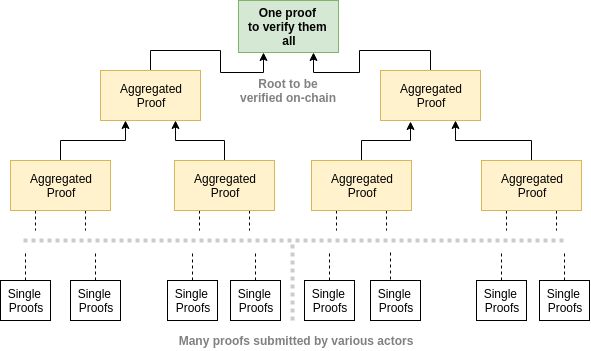# Recursive Zero-Knowledge Proofs

We present recursive Zero-Knowledge Proofs (ZKPs), where a proof attests to the validity of another proof. We show its benefits over standard non-recursive ZKPs and showcases its power by applying it to proving Fibonacci sequences.

# What is a recursive ZKP

Suppose Peggy wants to prove to Victor that she will spend next week in a park and she wants to do so using just one photo. She can do the following:

On day 1, she takes a photo in the park with a calendar showing the date.

On day 2, she takes another photo in the park with a calendar, while holding the photo taken from day 1.

On day 3, she takes another photo in the park with a calendar, while holding the photo taken from day 2.

The same procedure is repeated until day 7. Now she has a proof of her week-long trip in a single photo.

Akin to the figurative example above, in a recursive ZK proof, the proof attests to the validity of another proof, which validates another proof itself, and so on.

# Why do we need it

A recursive ZKP enjoys several salient advantages over a standard ZKP.

## Aggregation

Multiple proofs can be aggregated into a single proof. The single proof is only valid if all constituent proofs are valid, and it is much easier to verify. This is especially appealing when proofs are verified on a blockchain. Thousands of proofs can be compressed into a single proof, saving enormous cost to verify.Multiple proofs are merged into a single proof

## Parallel proof generation

Suppose that we want to prove that a batch of 1,000 transactions are valid in a rollup. Using standard ZKP, a prover generates a single proof to verify 1,000 transactions sequentially, a very time-consuming task.

Thanks to recursive ZKP, the prover can generate 1,000 proofs, one for each transaction. All proofs can be generated in parallel, since they are all independent, resulting a much smaller prover time. These individual transaction proofs can be recursively aggregated in a single proof as shown above.

## Incrementally verifiable computation (IVC)

Proving some types of computation is more efficient if the proof is incrementally updatable.

• Long computation: proving an excessively long computation takes huge amount of memory at the prover side. Some computation cannot even be fit in the memory, making proving it impossible.
• Evolving computation: for example, we want to prove the state of a blockchain but it is constantly growing. We compute a new proof that not only validates the new blocks, but also the existing proof itself.

We break the computation into smaller steps and prove them iteratively. Each step contains a proof , proving the current state of the computation. Using recursive ZKP (more specifically, IVC), a new proof can be generated for the next step by using the current step and its proof recursively. The proof update does not require recomputing from the very first step as in a standard ZKP, and is independent of the total length of the computation.

For example, we have the following computation for function F for i from 0 to n:

zᵢ is the public input and wᵢ the private input (i.e., witness). We want to efficiently prove the final output is zₙ if we start with z₀.

IVC achieves this by generating an initial proof 𝛑₀ (ΡF is the prover algorithm) and incrementally updating it at each step. For instance, 𝛑₂ not only proves F(z₁, w₁) = z₂, but also proves 𝛑₁ is valid as well. By induction, the final 𝛑ₙ proves all intermediate steps are correct.

# How does it work

On a high level, ZKP such as SNARKs can verify arbitrary computations. Since verifying a SNARK is a computation itself, SNARKs can verify other SNARK proofs. A recursive SNARK proof proves the existence of a previous valid proof.

Concretely, a computation has to be expressed in the form of a circuit for it to be proved by SNARKs. Recall that a verifier runs the verification algorithm with verification key, the proof, and the public input. Since the verification is a computation itself, it can expressed in a circuit. A proof on this computation/circuit certifies the validity of an inner proof, which may include another proof.

So far, we have explained how recursive SNARKs work in theory. In practice, verification is an intensive computation involving heavy cryptographic operations such as bilinear pairings. Many novel techniques such as cycle of elliptic curves have to be adopted for it to work efficiently. We won’t dwell on these practical issues in this short blog post.

# An Fibonacci example

We apply recursive SNARKs to Fibonacci sequence. The Fibonacci sequence is defined by the recurrence relation:

Peggy wants to convince Victor that a number is in the sequence, say, 55 (F₁₀). They use snarkyjs, a Typescript/Javascript framework for recursive SNARKs.

Line 3–38 define the recursive proof. We have the base cases fib0 at Line 11, and fib1 at line 18. The inductive case starts at Line 26. The proofs are recursively verified at Line 31 and 32, saying the proof for Fₙ is only valid if the proofs for Fₙ-₁ and Fₙ-₂ are both valid, and the formula is followed.

Line 41 compiles the circuit and retrieves the verification key.

Peggy iteratively generates proofs from Line 44 to 64.

Victor verifies the final proof using the verification key. It is worth noting he only needs the final proof and verifies it within seconds, regardless of the sequence index N at Line 50.

Victor may just recompute the whole sequence till N of 10 in this toy example and verify it instantly. The power of recursive SNARKs is more apparent when N is large, say 1,000,000,000,000. Victor can still verify the proof within seconds, exponentially faster than recalculating it.

--

--## sCrypt

sCrypt (https://scrypt.io) is a company with a mission to provide integrated on-chain smart contracting solutions on Bitcoin SV